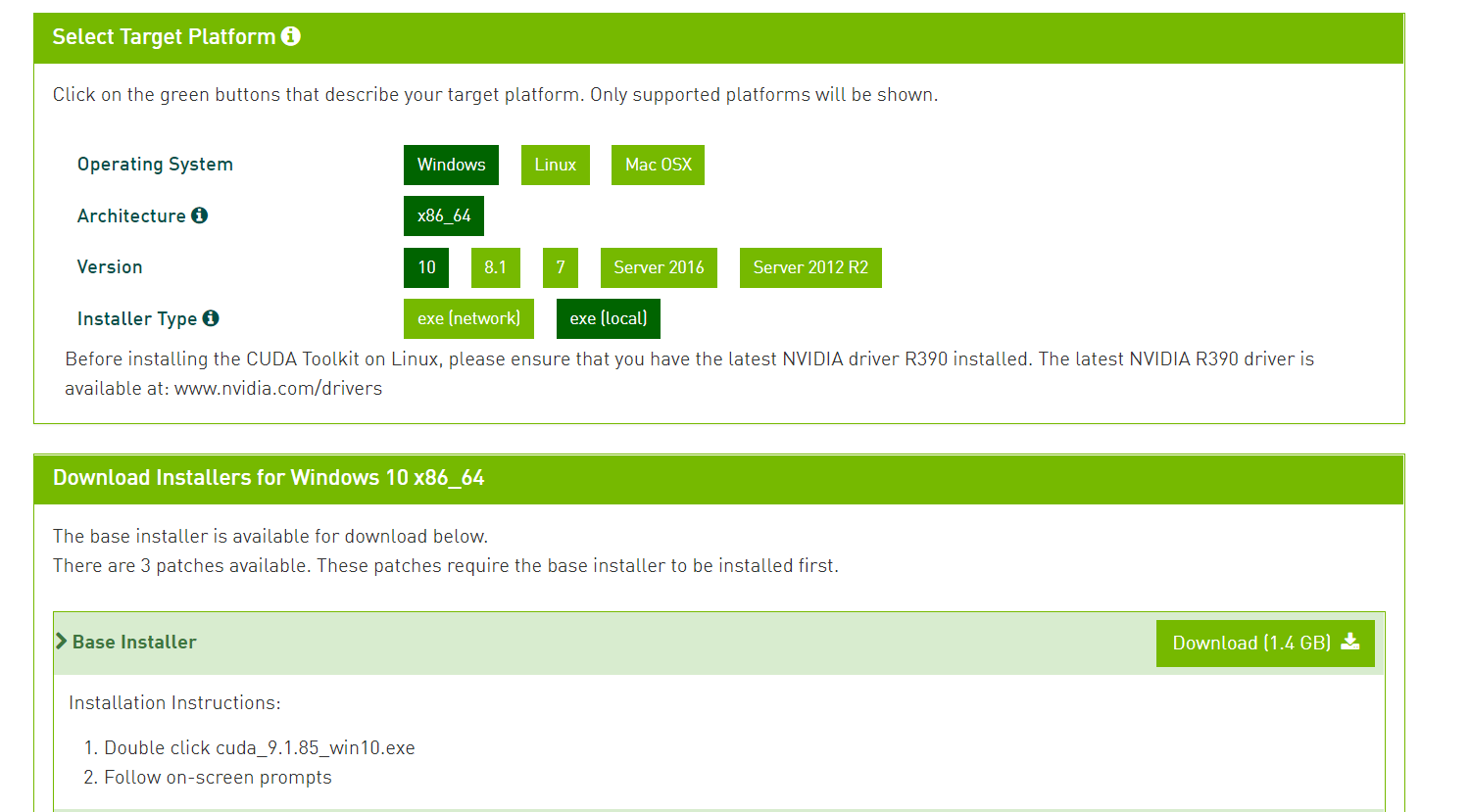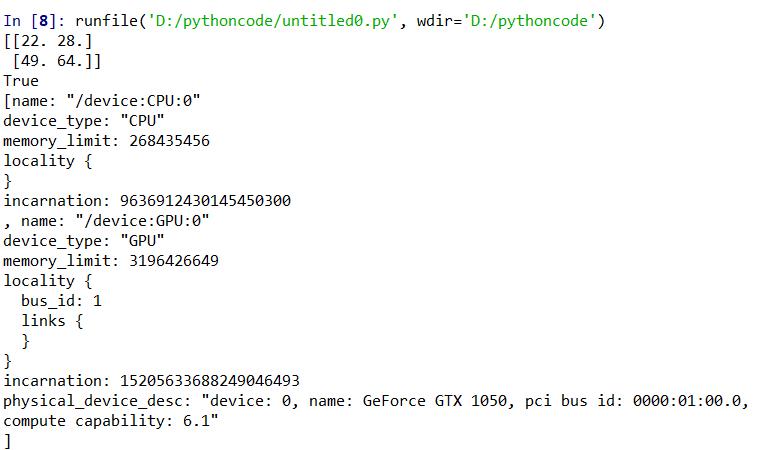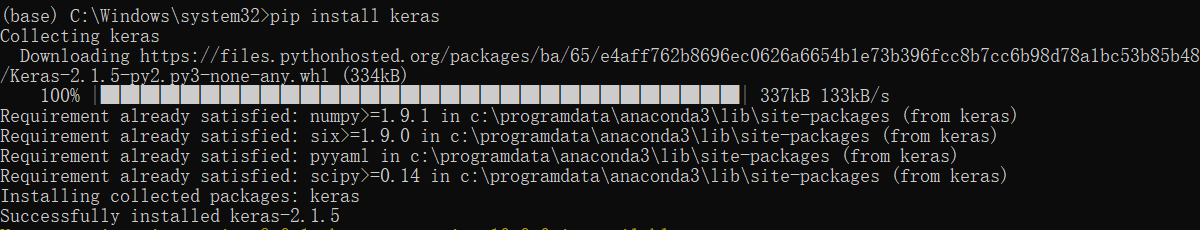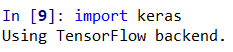# 安装环境：

• windows 10 64bit
• python 3.6

# 2、安装cuda

Tensorflow已经更新到1.7版本了，官网上说支持最新的cuda 9.X和cuDNN 7.X（结果被坑，后期详述），在Nvidia官网上下载最新的cuda和cuDNN。# 3、安装cuDNN# 4、安装tensorflow GPU版本

``````import tensorflow as tf
hello = tf.constant('Hello, TensorFlow!')
sess = tf.Session()
print(sess.run(hello))```

```

````ImportError: Could not find 'cudart64_90.dll'. TensorFlow requires that this DLL be installed in a directory that is named in your %PATH% environment variable. Download and install CUDA 9.0 from this URL: https://developer.nvidia.com/cuda-toolkit`

```

`pip uninstall tensorflow-gpu`

`pip install 文件存放路径\文件名.whl`

``````import tensorflow as tf
#Creates a graph.
a = tf.constant([1.0, 2.0, 3.0, 4.0, 5.0, 6.0], shape=[2, 3], name='a')
b = tf.constant([1.0, 2.0, 3.0, 4.0, 5.0, 6.0], shape=[3, 2], name='b')
c = tf.matmul(a, b)
#Creates a session with log_device_placement set to True.
sess = tf.InteractiveSession()
#Runs the op.
print(sess.run(c))

from tensorflow.python.client import device_lib as _device_lib
print(any((x.device_type == 'GPU') for x in _device_lib.list_local_devices()))
print(_device_lib.list_local_devices())```

```# 4、安装keras

`pip install keras``import keras`